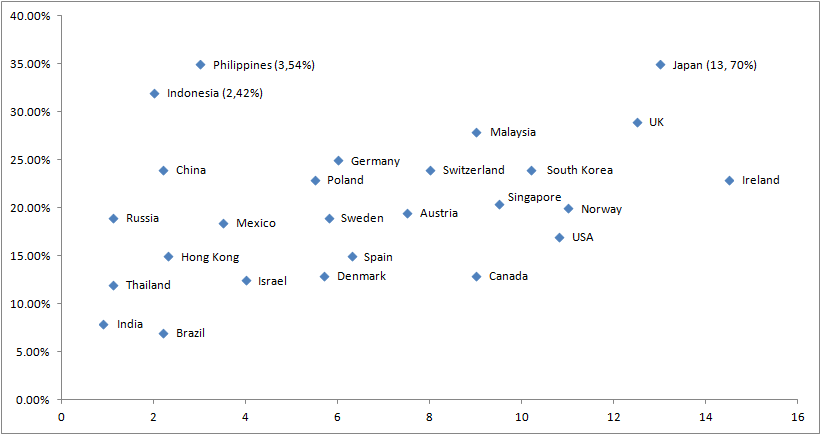### CAT 2008 Question Paper Question 30

Instructions

Directions for the next three questions:

Answer the following questions based on the information given below: Telecom operators get revenue from transfer of data and voice. Average revenue received from transfer of each unit of data is known as ARDT. In the diagram below, the revenue received form data transfer as percentage of total revenue received and the ARDT in US Dollars (USD) are given for various countries.

The X axis represents the ARDT values and the Y axis represents the % of revenue from data transfer as a percentage of the total revenue.Question 30

# It is expected that by 2010, revenue from data transfer as a percentage of total revenue will triple for India and double for Sweden. Assume that in 2010, the total revenue in India is twice that of Sweden and that the volume of data transfer is the same in both the countries. What is the percentage increase of ARDT in India if there is no change in ARDT in Sweden?

Solution

Revenue from data transfer as a percentage of total revenue will triple for India and double for Sweden.
Also the total revenue in India is twice that of Sweden.
Taking volume constant we have fir India  $$3*0.9*R_i = ARDT * vol$$
For Sweden  $$0.18*2*R_s = 6 * Vol$$
Since vol is constant and $$R_i = 2* R_s$$ we have ARDT = 9 .
Thus % increase in ARDT = 800%.